Courses

# Speed Control of Induction Machines Electrical Engineering (EE) Notes | EduRev

## Electrical Engineering (EE) : Speed Control of Induction Machines Electrical Engineering (EE) Notes | EduRev

The document Speed Control of Induction Machines Electrical Engineering (EE) Notes | EduRev is a part of the Electrical Engineering (EE) Course Electrical Machines for Electrical Engg..
All you need of Electrical Engineering (EE) at this link: Electrical Engineering (EE)

Speed control of Induction Machines

We have seen the speed torque characteristic of the machine. In the stable region of operation in the motoring mode, the curve is rather steep and goes from zero torque at synchronous speed to the stall torque at a value of slip s =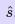. Normallymay be such that stall torque is about three times that of the rated operating torque of the machine, and hence may be about 0.3 or less. This means that in the entire loading range of the machine, the speed change is quite small. The machine speed is quite stiﬀ with respect to load changes. The entire speed variation is only in the range ns to (1 −)ns, ns being dependent on supply frequency and number of poles.

The foregoing discussion shows that the induction machine, when operating from mains is essentially a constant speed machine. Many industrial drives, typically for fan or pump applications, have typically constant speed requirements and hence the induction machine is ideally suited for these. However,the induction machine, especially the squirrel cage type, is quite rugged and has a simple construction. Therefore it is good candidate for variable speed applications if it can be achieved.

Speed control by changing applied voltage

From the torque equation of the induction machine given in eqn.17, we can see that the torque depends on the square of the applied voltage. The variation of speed torque curves with respect to the applied voltage is shown in ﬁg. 27. These curves show that the slip at maximum torqueremains same, while the value of stall torque comes down with decrease in applied voltage. The speed range for stable operation remains the same.

Further, we also note that the starting torque is also lower at lower voltages. Thus, even if a given voltage level is suﬃcient for achieving the running torque, the machine may not start. This method of trying to control the speed is best suited for loads that require very little starting torque, but their torque requirement may increase with speed.

Figure 27 also shows a load torque characteristic — one that is typical of a fan type of load. In a fan (blower) type of load,the variation of torque with speed is such that T ∝ ω2.

Here one can see that it may be possible to run the motor to lower speeds within the range ns to (1 −)ns. Further, since the load torque at zero speed is zero, the machine can start even at reduced voltages. This will not be possible with constant torque type of loads.

One may note that if the applied voltage is reduced, the voltage across the magnetising branch also comes down. This in turn means that the magnetizing current and hence ﬂux level are reduced. Reduction in the ﬂux level in the machine impairs torque production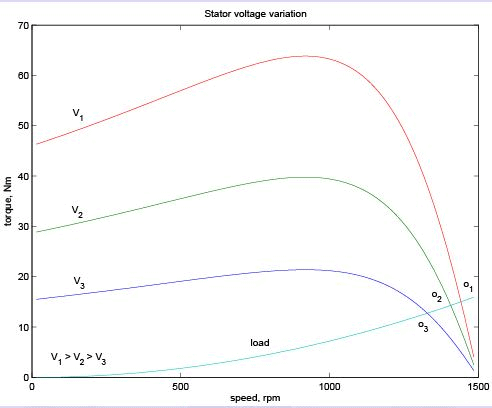Figure 27: Speed-torque curves: voltage variation

(recall explantions on torque production), which is primarily the explanation for ﬁg. 27. If, however, the machine is running under lightly loaded conditions, then operating under rated ﬂux levels is not required. Under such conditions, reduction in magnetizing current improves the power factor of operation. Some amount of energy saving may also be achieved.

Voltage control may be achieved by adding series resistors (a lossy, ineﬃcient proposition), or a series inductor / autotransformer (a bulky solution) or a more modern solution using semiconductor devices. A typical solid state circuit used for this purpose is the AC voltage controller or AC chopper. Another use of voltage control is in the so-called ‘soft-start’ of the machine. This is discussed in the section on starting methods.

Rotor resistance control

The reader may recall from eqn.17 the expression for the torque of the induction machine.
Clearly, it is dependent on the rotor resistance. Further, eqn.19 shows that the maximum value is independent of the rotor resistance. The slip at maximum torque eqn.18 is dependent on the rotor resistance. Therefore, we may expect that if the rotor resistance is changed, the maximum torque point shifts to higher slip values, while retaining a constant torque. Figure 28 shows a family of torque-speed characteristic obtained by changing the rotor resistance.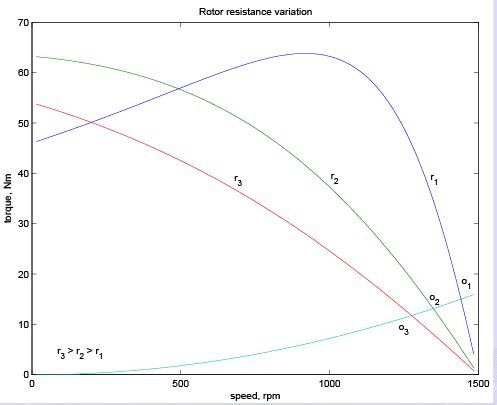Figure 28: Speed-torque curves : rotor resistance variation

Note that while the maximum torque and synchronous speed remain constant, the slip at which maximum torque occurs increases with increase in rotor resistance, and so does the starting torque. whether the load is of constant torque type or fan-type, it is evident that the speed control range is more with this method. Further, rotor resistance control could also be used as a means of generating high starting torque.

For all its advantages, the scheme has two serious drawbacks. Firstly, in order to vary the rotor resistance, it is necessary to connect external variable resistors (winding resistance itself cannot be changed). This, therefore necessitates a slip-ring machine, since only in that case rotor terminals are available outside. For cage rotor machines, there are no rotor terminals. Secondly, the method is not very eﬃcient since the additional resistance and operation at high slips entails dissipation.

The resistors connected to the slip-ring brushes should have good power dissipation capability. Water based rheostats may be used for this. A ‘solid-state’ alternative to a rheostat is a chopper controlled resistance where the duty ratio control of of the chopper presents a variable resistance load to the rotor of the induction machine.

The power drawn from the rotor terminals could be spent more usefully. Apart from using the heat generated in meaning full ways, the slip ring output could be connected to another induction machine. The stator of the second machine would carry slip frequency currents of the ﬁrst machine which would generate some useful mechanical power. A still better option would be to mechanically couple the shafts of the two machines together. This sort of a connection is called cascade connection and it gives some measure of speed control as shown below.

Let the frequency of supply given to the ﬁrst machine be f, its number poles be p1, and its slip of operation be s1 . Let f2 , p2 and s2 be the corresponding quantities for the second machine. The frequency of currents ﬂowing in the rotor of the ﬁrst machine and hence in the stator of the second machine is s1f1 . Therefore f2 = s1 f1. Since the machines are coupled at the shaft, the speed of the rotor is common for both. Hence, if n is the speed of the rotor in radians,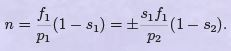(20)
Note that while giving the rotor output of the ﬁrst machine to the stator of the second, the resultant stator mmf of the second machine may set up an air-gap ﬂux which rotates in the same direction as that of the rotor, or opposes it. this results in values for speed as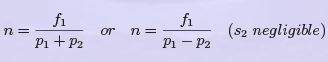(21)
The latter expression is for the case where the second machine is connected in opposite phase sequence to the ﬁrst. The cascade connected system can therefore run at two possible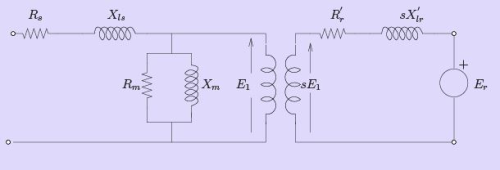Figure 29: Generalized rotor control

speeds.

Speed control through rotor terminals can be considered in a much more general way.
Consider the induction machine equivalent circuit of ﬁg. 29, where the rotor circuit has been terminated with a voltage source Er .

If the rotor terminals are shorted, it behaves like a normal induction machine. This is equivalent to saying that across the rotor terminals a voltage source of zero magnitude is connected. Diﬀerent situations could then be considered if this voltage source Er had a non-zero magnitude. Let the power consumed by that source be Pr . Then considering the rotor side circuit power dissipation per phase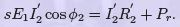(22) Clearly now, the value of s can be changed by the value of Pr . For Pr = 0, the machine is like a normal machine with a short circuited rotor. As Pbecomes positive, for all other circuit conditions remaining constant, s increases or in the other words, speed reduces. As Pr becomes negative,the right hand side of the equation and hence the slip decreases. The physical interpretation is that we now have an active source connected on the rotor side which is able to supply part of the rotor copper losses. When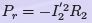the entire copper loss is supplied by the external source. The RHS and hence the slip is zero. This corresponds to operation at synchronous speed. In general the circuitry connected to the rotor may not be a simple resistor or a machine but a power electronic circuit which can process this power requirement. This circuit may drive a machine or recover power back to the mains. Such circuits are called static kramer drives.

Pole changing schemes

Sometimes induction machines have a special stator winding capable of being externally connected to form two diﬀerent number of pole numbers. Since the synchronous speed of the induction machine is given by ns = fs/p (in rev./s) where p is the number of pole pairs, this would correspond to changing the synchronous speed. With the slip now corresponding to the new synchronous speed, the operating speed is changed. This method of speed control is a stepped variation and generally restricted to two steps.

If the changes in stator winding connections are made so that the air gap ﬂux remains constant, then at any winding connection, the same maximum torque is achievable.

Such winding arrangements are therefore referred to as constant-torque connections. If however such connection changes result in air gap ﬂux changes that are inversely proportional to the synchronous speeds, then such connections are called constant-horsepower type.

The following ﬁgure serves to illustrate the basic principle. Consider a magnetic pole structure consisting of four pole faces A, B, C, D as shown in ﬁg. 30.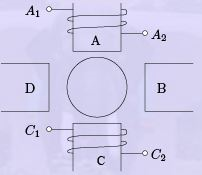Figure 30: Pole arrangement

Coils are wound on A & C in the directions shown. The two coils on A & C may be connected in series in two diﬀerent ways — A2 may be connected to C1 or C2. A1 with the other terminal at C then form the terminals of the overall combination. Thus two connections result as shown in ﬁg. 31 (a) & (b).

Now, for a given direction of current ﬂow at terminal A1, say into terminal A1, the ﬂux directions within the poles are shown in the ﬁgures. In case (a), the ﬂux lines are out of the pole A (seen from the rotor) for and into pole C, thus establishing a two-pole structure. In case (b) however, the ﬂux lines are out of the poles in A & C. The ﬂux lines will be then have to complete the circuit by ﬂowing into the pole structures on the sides. If, when seen from the rotor, the pole emanating ﬂux lines is considered as north pole and the pole into which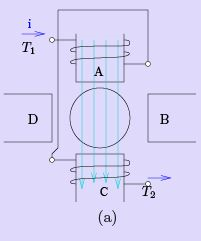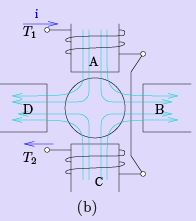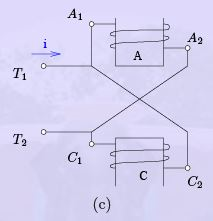Figure 31: Pole Changing: Various connections

they enter is termed as south, then the pole conﬁgurations produced by these connections is a two-pole arrangement in ﬁg. 31(a) and a four-pole arrangement in ﬁg. 31(b).

Thus by changing the terminal connections we get either a two pole air-gap ﬁeld or a fourpole ﬁeld. In an induction machine this would correspond to a synchronous speed reduction in half from case (a) to case (b). Further note that irrespective of the connection, the applied voltage is balanced by the series addition of induced emfs in two coils. Therefore the air-gap ﬂux in both cases is the same. Cases (a) and (b) therefore form a pair of constant torque connections.

Consider, on the other hand a connection as shown in the ﬁg. 31(c). The terminals T1 and T2 are where the input excitation is given. Note that current direction in the coils now resembles that of case (b), and hence this would result in a four-pole structure. However, in ﬁg. 31(c), there is only one coil induced emf to balance the applied voltage. Therefore ﬂux in case (c) would therefore be halved compared to that of case (b) (or case (a), for that matter). Cases (a) and (c) therefore form a pair of constant horse-power connections.

It is important to note that in generating a diﬀerent pole numbers, the current through one coil (out of two, coil C in this case) is reversed. In the case of a three phase machine, the following example serves to explain this. Let the machine have coils connected as shown [C1 − C6] as shown in ﬁg. 32.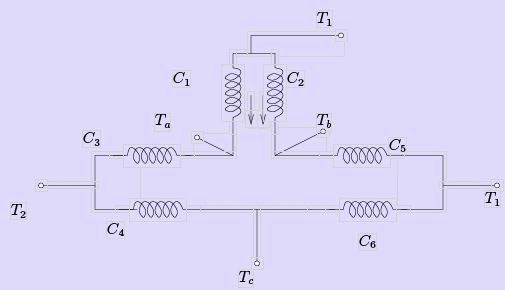Figure 32: Pole change example: three phase

The current directions shown in C1 & C2 correspond to the case where T1 , T2, T3 are supplied with three phase excitation and Ta, Tb & Tc are shorted to each other (STAR point). The applied voltage must be balanced by induced emf in one coil only (C1 & C2 are parallel). If however the excitation is given to Ta , Tb& Tc with T1 , T2, T3 open, then current through one of the coils (C1 & C2) would reverse. Thus the eﬀective number of poles would increase, thereby bringing down the speed. The other coils also face similar conditions.

Stator frequency control

The expression for the synchronous speed indicates that by changing the stator frequency also it can be changed. This can be achieved by using power electronic circuits called inverters which convert dc to ac of desired frequency. Depending on the type of control scheme of the inverter, the ac generated may be variable-frequency-ﬁxed-amplitude or variable-frequencyvariable-amplitude type. Power electronic control achieves smooth variation of voltage and frequency of the ac output. This when fed to the machine is capable of running at a controlled speed. However, consider the equation for the induced emf in the induction machine.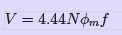(23)

where N is the number of the turns per phase, φm is the peak ﬂux in the air gap and f is the frequency. Note that in order to reduce the speed, frequency has to be reduced. If the frequency is reduced while the voltage is kept constant, thereby requiring the amplitude of induced emf to remain the same, ﬂux has to increase. This is not advisable since the machine likely to enter deep saturation. If this is to be avoided, then ﬂux level must be maintained constant which implies that voltage must be reduced along with frequency. The ratio is held constant in order to maintain the ﬂux level for maximum torque capability.

Actually, it is the voltage across the magnetizing branch of the exact equivalent circuit that must be maintained constant, for it is that which determines the induced emf. Under conditions where the stator voltage drop is negligible compared the applied voltage, eqn. 23 is valid.

In this mode of operation, the voltage across the magnetizing inductance in the ’exact’ equivalent circuit reduces in amplitude with reduction in frequency and so does the inductive reactance. This implies that the current through the inductance and the ﬂux in the machine remains constant. The speed torque characteristics at any frequency may be estimated as before. There is one curve for every excitation frequency considered corresponding to every value of synchronous speed. The curves are shown below. It may be seen that the maximum torque remains constant.

This may be seen mathematically as follows. If E is the voltage across the magnetizing branch and f is the frequency of excitation, then E = kf , where k is the constant of proportionality. If ω = 2πf , the developed torque is given by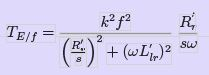(24)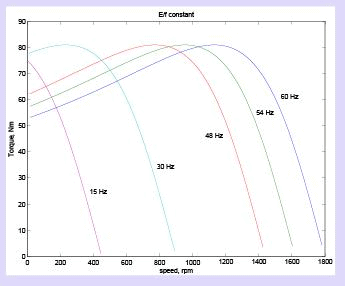Figure 33: Torque-speed curves with E /f held constant If this equation is diﬀerentiated with repsect to s and equated to zero to ﬁnd the slip at maximum torque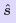, we get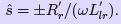The maximum torque is obtained by substituting this value into eqn. 24.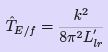(25)
Equation 25 shows that this maximum value is indepedent of the frequency. Furtherω is independent of frequency. This means that the maximum torque always occurs at a speed lower than synchronous speed by a ﬁxed diﬀerence, independent of frequency. The overall eﬀect is an apparent shift of the torque-speed characteristic as shown in ﬁg. 33.

Though this is the aim, E is an internal voltage which is not accessible. It is only the terminal voltage V which we have access to and can control. For a ﬁxed V , E changes with operating slip (rotor branch impedance changes) and further due to the stator impedance drop. Thus if we approximate E /f as V /f , the resulting torque-speed characteristic shown in ﬁg. 34 is far from desirable.

At low frequencies and hence low voltages the curves show a considerable reduction in peak torque. At low frequencies ( and hence at low voltages) the drop across the stator impedance prevents suﬃcient voltage availability. Therefore, in order to maintain suﬃcient torque at low frequencies, a voltage more than proportional needs to be given at low speeds.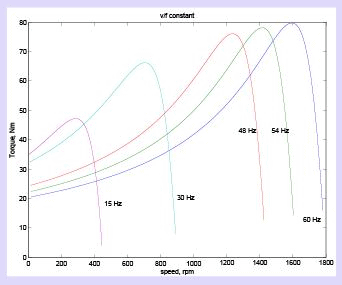Figure 34: Torque-speed curves with V /f constant

Another component of compensation that needs to be given is due to operating slip. With these two components, therefore, the ratio of applied voltage to frequency is not a constant but is a curve such as that shown in ﬁg. 35

With this kind of control, it is possible to get a good starting torque and steady state performance. However, under dynamic conditions, this control is insuﬃcient. Advanced control techniques such as ﬁeld- oriented control (vector control) or direct torque control (DTC) are necessary.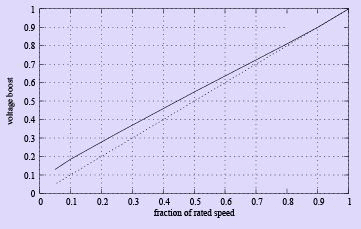Figure 35: Voltage boost required for V /f control

Offer running on EduRev: Apply code STAYHOME200 to get INR 200 off on our premium plan EduRev Infinity!

## Electrical Machines for Electrical Engg.

22 videos|41 docs|26 tests

,

,

,

,

,

,

,

,

,

,

,

,

,

,

,

,

,

,

,

,

,

;